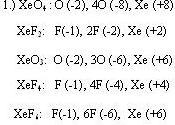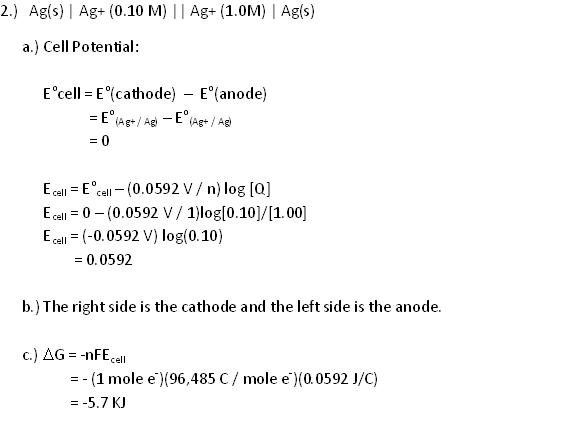# HW Solutions #1

## Redox Basics

1.) Xenon forms several nonionic compounds with F and O. Give the oxidation number of the central Xe atom in each of the following compounds: XeO4, XeF2, XeO3, XeF4, and XeF6.2.) Xenon can also form a number of ionic compounds, including CsXeF7, and Cs2XeF8. What are the ions in each compound? What is the formal charge and oxidation number of Xe in each ion containing Xe?

a.) CsXeF7 → Cs+ + XeF7-. Xe has +6 oxidation state and -1 formal charge.

b.) CsXeF8 → 2Cs+ + XeF82-. Xe has +6 oxidation state and -2 formal charge.

4.) What is the oxidation number of nitrogen in each of the following ions or molecules: NH3, N2H4, NO, NO2, NO2-, NO3-?

NH3, x + 3(1) = 0, x = -3

N2H4, 2x + 4(1) = 0, 2x = -4, x = -2

NO, x + -2 = 0, x = 2

NO2, x + 2(-2) = 0, x = 4

NO2-, x + 2(-2) = -1, x = 3

NO3-, x + 3(-2) = -1, x = 5

5.) What is the oxidation number of platinum in the complex ion PtCl42-?

Pt + 4(-1) = -2

Pt – 4 = -2

Pt = +2

6.) Assign oxidation numbers to the atoms in the following chemical species:

1. gold, Au
2. iodine, I2
3. barium chloride, BaCl2
4. ethane, C2H6
5. stannous oxide, SnO
6. stannic oxide, SnO2
7. nitrous oxide, N2O
8. phosphorus pentoxide, P2O5
9. magnesium hydroxide, Mg(OH)2
10. sulfurous acid, H2SO3
11. telluric acid, H6TeO6
12. hypochlorous acid, HClO
13. perchloric acid, HCIO4
14. dichromate, Cr2O72-
15. cyanide, CN-7.) What is the oxidation number of the underlined element in each ion or molecule: VO2+, P2O74-, PH3, KNO2, H2O2, LiH, Mg3N2, NF3, ICl5, Ag(NH3)2+?

VO2+: 4+ P2O74-:5+ PH3: 3- KNO2: 3+ H2O2: 1-
LiH: 1- Mg3N2:2+ NF3: 3+ ICl5:5+ Ag(NH3)2+: 2+

## Redox Equations

8.) When the equation

MnO2 + I- + H+ → Mn2+ + I2 + H2O

is balanced, what is the net charge on each side of the equation? What element is oxidized? What element is reduced?

Mn02 + I- + H+ → Mn2+ I2+ H20

Mn02 → Mn2+

oxidation state of Mn goes from 4 to 2

Its reduced

I- → I2

Oxidation state I goes from - 1 to 0

Hence oxidized

H is a spectator ion , oxidation state is 1 on both the reactant and product side. for redox reaction to occur there has to be a gain or loss of electrons

8b: Multiple Choice: In the following reaction, which element that is oxidized and which is the oxidizing agent.

2 Al + 3 Br2 → 2 AlBr3

(a) aluminum…..aluminum
(b) bromine…..bromine
(c) aluminum…..bromine
(d) bromine…..aluminum

2Al + 3Br2 → 2AlBr3

Oxidate of Al = 0, Oxiditaion state of Br2 = 0 ( pure form as solid metal and Gas)

2Al + 3Br2 → 2AlBr3 [for Al 2[x + 3(-1) ] = 0, 2x = 6, x = 3 for Al

0 0 Al = 3 and Br2 = -1

Al is Oxidized and Br is reduced

Al is the reducing agent and Br2 is the oxidizing agent

9) For each of the following reactions, list the substance reduced, the substance oxidized, the reducing agent, and the oxidizing agent.

1. 6H+ + MnO4- + 5SO32- → 5SO42- + 2Mn2+ + 3H2O
2. 8H+ + Cr2O72- + 6HI → 2Cr3+ + 312 + 7H2O
3. 3Cl2 + 6OH- → CIO3- + 5Cl- + 3H2O

a.) Mn7+ is reduced to Mn2+. S4+ is oxidized to S6+. MnO4- is the oxidizing agent. SO32- is the reducing agent.

b.) Cr6+ is reduced to Cr3+. I- is oxidized to I0. Cr2O72- is the oxidizing agent. HI is the reducing agent.

c.) Cl2 is both oxidized and reduced, so acts as the oxidizing and reducing agent.

10.) Phosphine, PH3, is a colorless, highly toxic gas that smells like rotten Fish and is produced in small amounts when animal and vegetable matter decay in moist situations such as damp graveyards, Traces of P2H4 are produced simultaneously and cause the PH3 to ignite in air to give pale, flickering lights commonly called “corpse candles" or "will-o’-the-wisps." In the laboratory, phosphine can be prepared by adding water to calcium phosphide. Write a balanced equation for the reaction. Assign oxidation numbers to each of the elements present.

Ca3P2 + 6 H2O → 3 Ca(OH)2 + 2 PH3

Oxidation number Ca +2 P -3 H +1 O -2

11.) Balance the reaction

MnO4- + H+ + H2S → Mn2+ + H2O + S

What is the oxidation number of Mn in MnO4-? What element is oxidized? What element is the oxidizing agent? Is this latter element oxidized or reduced?

Step 1:Write half reactions separately by identifying oxidation and reduction species

Eq.1 MnO4- Mn2+

Mn in Mn2+ has an oxidation state = +2

Oxidation State Change: +7 +2

Eq. 2 H2S S

S is being oxidized (loss of 2 electrons)

Step 2: Balance elements other than H or O, if any

Eq.1 MnO4- Mn2+

Both sides consist of 1 Mn, therefore, Mn is balanced

Eq. 2 H2S S

Both sides consist of 1 S, therefore, S is balanced

Step 3: Balance O by adding H2O molecules

Eq.1 (old) MnO4- Mn2+

Eq. 2 (old) H2S S

Eq. 2 (new) H2S S

No oxygen in Eq. 2

Step 4: Balance H by adding H+ molecules

Eq.1 (old) MnO4- Mn2+ + 4H2O

Eq.1 (new) 8H+ + MnO4- Mn2+ + 4H2O

Eq. 2 (old) H2S S

Eq. 2 (new) H2S S + 2H+

Step 5: Balance charge by adding electrons to more positive side

Eq.1 (old) 8H+ + MnO4- Mn2+ + 4H2O

Total charge on reactants side 8H+ + MnO4- : 8(+1) + 1(-1) = +7

Total charge on products side Mn2+ + 4H2O: 1(+2) + 4(0) = +2

Reactants are more positive therefore, 5 electrons will be added left

Eq.1 (new) 5e- + 8H+ + MnO4- Mn2+ + 4H2O

Eq. 2 (old) H2S S + 2H+

Total charge on reactants side H2S: 1(0) = 0

Total charge on products side S + 2H+: 1(0) + 2(+1) = +2

Product side is more positive, therefore 2 electrons will be added to the right

Eq. 2 (new) H2S S + 2H+ + 2e-

Step 6: Cancel electrons by multiplying the whole equation by a whole number

Eq.1 (old) 5e- + 8H+ + MnO4- Mn2+ + 4H2O

Eq.1 (new) [5e- + 8H+ + MnO4- Mn2+ + 4H2O] x 2

Eq.1 (new) 10e- + 16H+ + 2MnO4- 2Mn2+ + 8H2O

Eq. 2 (old) H2S S + 2H+ + 2e-

Eq. 2 (new) [H2S S + 2H+ + 2e-] x 5

Eq. 2 (new) 5H2S 5S + 10H+ + 10e-

With 10 electrons on both sides, electrons can be canceled!

Step 7: Add half reactions and cancel common terms on both sides

Eq.1 (old) 10e- + 16H+ + 2MnO4- 2Mn2+ + 8H2O

Eq. 2 (old) 5H2S 5S + 10H+ + 10e-

Adding both half-reactions together and canceling 10 electrons on both sides gives:

16H+ + 2MnO4- + 5H2S 2Mn2+ + 8H2O + 5S + 10H+

Canceling H+ that are common to both sides of the equation gives the final balanced equation:

6H+ + 2MnO4- + 5H2S 2Mn2+ + 8H2O + 5S

What is the oxidation number of Mn in MnO4-?

MnO4-

Mn + 4(-2) = -1

Mn – 8 = -1

Mn = +7

What element is oxidized?

S is oxidized (refer to Step 1 above for explanation)

What element is the oxidizing agent?

Mn is being reduced and thus, it is the oxidizing agent

## Electrochemistry

1.) A standard Cl2 | Cl- half-cell has been What is ΔG (in joules) for the spontaneous coupled to a Cl2 (1.00 atm) l Cl- (0.010M) half-cell. What is the cell voltage? Determine ΔG (at room temperature) for this reaction.2. The following cell

Ag | Ag+(0.10M) || Ag+(1.0M) | Ag

is a concentration cell and is capable of electrical work.

(a) What is the cell potential?

(b) Which side of the cell is the cathode and which is the anode?

(c) What is the ΔG (in joules) for the spontaneous cell reaction?3. Two copper electrodes are placed in two copper sulfate solutions of equal concentration and connected to form a concentration cell. What is the cell voltage?

Because the half reactions are the same, there will be no change. The cell voltage will be zero. This can be proved with the Nernst Equation.

E = Eo - (0.0592V/2)log(Cu2+dilute/Cu2+concentrated)
Eo = 0 because half reactions are the same.
Cu2+dilute/Cu2+concentrated = 1 because concentrations are the same.
log(1) = 0 so the equation becomes:
E = 0 - (0.0592V/2)(0)
E = 0

One of the solutions is diluted until the concentration of copper ions is one-fifth its original value. What is the cell voltage after dilution?

E can be calculated using the Nernst equation:

E = Eo - (0.0592V/2)log(Cu2+dilute/Cu2+concentrated)
Eo = 0 because half reactions are the same.
Cu2+dilute/Cu2+concentrated = (1/5)/(1) = 0.2/1 = 0.2
log(0.2) = -0.69897 so the equation becomes:
E = 0 - (0.0592V/2)(-0.69897)
E = +0.021

## Spontaneity

6. Assuming unit activities for all substances, determine which of the following reactions will be spontaneous:

These can be figured out using the Activity Series or a Table of Standard Electrode Potentials

a. Zn + Mg2+ → Zn2+ + Mg Non-Spontaneous.Mg is a stronger reducer than Zn

b. Fe + Cl2 → Fe2+ + 2Cl- Spontaneous. Fe is a stronger reducer than Cl-

c. 4Ag + O2 + 4H+ → 4Ag+ +2H2O Spontaneous. Ag is a stronger reducer than H20

d. 2AgCl → 2Ag + Cl2 Non-Spontaneous. Ag is a stronger reducer than Cl-

## Half-Cell Potentials

7.) What are the potentials for the following cells or half-cells?

a) Eo for the cell: Zn(s) | Zn2+|| Cu2+ l Cu(s)

b) E for the half-cell: Zn(s) l Zn2+(0.001M)

c) E for the half-cell: Cu2+ (1O-36M) | Cu(s)

a.) Eo = E(right)-E(left) = E(Cu2+/Cu)-E(Zn2+/Zn) = 0.340 - (-0.763) = 1.103 V

b.) E = Eo(Zn2+/Zn) - 0.0592/2 log(1/0.001M) = -0.852 V

c.) E = Eo(Cu2+/Cu) - 0.0592/2 log (1/10-36 M) = -0.726 V

8.) What are the standard potentials, Eo, for the following half-cells?

1. S2- | CuS (s) | Cu(s)
2. NH3(aq), Zn(NH3)42+ | Zn(s)

Neglect the ligands:

a.) Cu2+ (aq) + 2e- Cu (S)

b.) Zn2+ (aq) + 2e- Zn (s)

Eq.1 (new) MnO4- → Mn2+ + 4H2O

S in H2S has an oxidation state = -2

S in S has an oxidation state = 0

Oxidation State Change: -2 0

Mn is being reduced (gains 5 electrons)

Mn in MnO4- has an oxidation state = +7